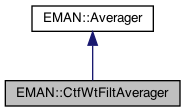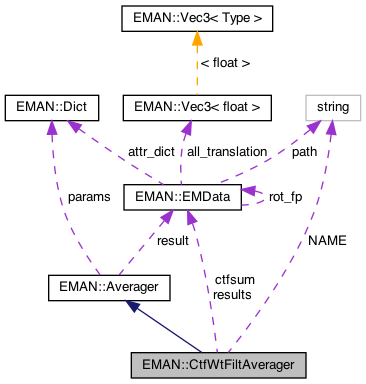EMAN2
EMAN::CtfWtFiltAverager Class Reference

`#include <averager.h>`

Inheritance diagram for EMAN::CtfWtFiltAverager:[legend]
Collaboration diagram for EMAN::CtfWtFiltAverager:[legend]

## Public Member Functions

CtfWtFiltAverager ()

To add an image to the Averager. More...

EMDatafinish ()
Finish up the averaging and return the result. More...

string get_name () const
Get the Averager's name. More...

string get_desc () const

void set_params (const Dict &new_params)
Set the Averager parameters using a key/value dictionary. More...Public Member Functions inherited from EMAN::Averager
Averager ()

virtual ~Averager ()

virtual void add_image_list (const vector< EMData * > &images)
To add multiple images to the Averager. More...

virtual void mult (const float &s)
Multiply the result image by some floating point constant This is useful when weighting the input images prior to calling add_image - a situation where it is likely you want to divide by the sum of the weights. More...

virtual TypeDict get_param_types () const
Get Averager parameter information in a dictionary. More...

## Static Public Member Functions

static AveragerNEW ()

## Static Public Attributes

static const string NAME = "ctf.weight.autofilt"

## Protected Attributes

EMDataresults 

EMDatactfsum 

int nimg 

int eoProtected Attributes inherited from EMAN::Averager
Dict params

EMDataresult

## Detailed Description

Definition at line 524 of file averager.h.

## ◆ CtfWtFiltAverager()

 CtfWtFiltAverager::CtfWtFiltAverager ( )

Definition at line 1343 of file averager.cpp.

1344{
1345 nimg=0;
1346 nimg=0;
1347 eo=-1;
1348}

References eo, and nimg.

Referenced by NEW().

## Member Function Documentation

 void CtfWtFiltAverager::add_image ( EMData * image )
virtual

To add an image to the Averager.

This image will be averaged in this function.

Parameters
 image The image to be averaged.

Reimplemented from EMAN::Averager.

Definition at line 1351 of file averager.cpp.

1352{
1353 if (!image) {
1354 return;
1355 }
1356
1357
1358
1359 EMData *fft=image->do_fft();
1360
1361 if (nimg >= 1 && !EMUtil::is_same_size(fft, results)) {
1362 LOGERR("%s Averager can only process images of the same size", get_name().c_str());
1363 return;
1364 }
1365
1366 if (eo==-1) {
1368 results->to_zero();
1370 results->to_zero();
1371 eo=1;
1372 }
1373
1374 eo^=1;
1375 nimg[eo]++;
1376
1377
1378 EMData *ctfi = results-> copy();
1379 if (image->has_attr("ctf")) {
1380 Ctf *ctf = (Ctf *)image->get_attr("ctf");
1381
1382 float b=ctf->bfactor;
1383 ctf->bfactor=0; // no B-factor used in weight, not strictly threadsafe, but shouldn't be a problem
1386 delete ctf;
1387 }
1388 else {
1389 ctfi->to_one();
1390 }
1391
1392 float *outd = results[eo]->get_data();
1393 float *ind = fft->get_data();
1394 float *ctfd = ctfi->get_data();
1395
1396 size_t sz=ctfi->get_xsize()*ctfi->get_ysize();
1397 for (size_t i = 0; i < sz; i+=2) {
1398
1399 // CTF weight
1400 outd[i]+=ind[i]*ctfd[i];
1401 outd[i+1]+=ind[i+1]*ctfd[i];
1402 }
1403
1404 if (nimg[eo]==1) {
1406 ctfsum[eo]->to_zero();
1407 ctfsum[eo]->add(0.1); // we start with a value of 0.1 rather than zero to empirically help with situations where the data is incomplete
1408 }
1410
1411 delete fft;
1412 delete ctfi;
1413}
EMData * ctfsum
Definition: averager.h:557
string get_name() const
Get the Averager's name.
Definition: averager.h:532
EMData * results
Definition: averager.h:556
Ctf is the base class for all CTF model.
Definition: ctf.h:60
virtual void compute_2d_complex(EMData *img, CtfType t, XYData *struct_factor=0)=0
float bfactor
Definition: ctf.h:85
@ CTF_INTEN
Definition: ctf.h:74
EMData stores an image's data and defines core image processing routines.
Definition: emdata.h:82
static bool is_same_size(const EMData *image1, const EMData *image2)
Check whether two EMData images are of the same size.
Definition: emutil.cpp:1224
EMData * copy() const
This file is a part of "emdata.h", to use functions in this file, you should "#include "emdata....
#define LOGERR
Definition: log.h:51

## ◆ finish()

 EMData * CtfWtFiltAverager::finish ( )
virtual

Finish up the averaging and return the result.

Returns
The averaged image.

Implements EMAN::Averager.

Definition at line 1415 of file averager.cpp.

1416{
1417 if (nimg==0 && nimg==0) return NULL; // no images
1418 // Only a single image, so we just return it. No way to filter
1419 if (nimg==0) {
1420 EMData *ret=results->do_ift();
1421 delete results;
1422 delete results;
1423 delete ctfsum;
1424 return ret;
1425 }
1426
1427 int nx=results->get_xsize();
1428 int ny=results->get_ysize();
1429
1430 for (int k=0; k<2; k++) {
1431 float *outd=results[k]->get_data();
1432 float *ctfsd=ctfsum[k]->get_data();
1433 for (int j=0; j<ny; j++) {
1434 for (int i=0; i<nx; i+=2) {
1435 size_t ii=i+j*nx;
1436 outd[ii]/=ctfsd[ii];
1437 outd[ii+1]/=ctfsd[ii];
1438 }
1439 }
1440 results[k]->update();
1442 results->set_attr("ctf_wiener_filtered",1);
1443 }
1444
1445 // compute the Wiener filter from the FSC
1446 std::vector<float> fsc=results->calc_fourier_shell_correlation(results);
1447 int third=fsc.size()/3;
1448 for (int i=third; i<third*2; i++) {
1449 if (fsc[i]>=.9999) fsc[i]=.9999;
1450 if (fsc[i]<.001) fsc[i]=.001;
1451 float snr=2.0*fsc[i]/(1.0-fsc[i]); // convert the FSC to SNR and multiply by 2
1452 fsc[i]=snr/(snr+1.0); // to give us the FSC of the combined average, which is also a Wiener filter (depending on whether SNR is squared)
1453 }
1454
1455
1457
1458 float c;
1459 for (int j=-ny/2; j<ny/2; j++) {
1460 for (int i=0; i<nx/2; i++) {
1461 int r=(int)Util::hypot_fast(i,j);
1462 if (r>=third) c=0.0;
1463 else c=fsc[third+r];
1464 results->set_complex_at(i,j,results->get_complex_at(i,j)*c);
1465 }
1466 }
1467
1468 EMData *ret=results->do_ift();
1469 ret->set_attr("ptcl_repr",nimg+nimg);
1470
1471/* snrsum->write_image("snr.hdf",-1);
1472 result->write_image("avg.hdf",-1);*/
1473
1474 delete ctfsum;
1475 delete ctfsum;
1476 delete results;
1477 delete results;
1478 results=results=NULL;
1479 return ret;
1480}
static float hypot_fast(int x, int y)
Euclidean distance in 2D for integers computed fast using a cached lookup table.
Definition: util.cpp:742
std::complex< float > get_complex_at(const int &x, const int &y) const
Get complex<float> value at x,y.

References ctfsum, get_complex_at(), EMAN::Util::hypot_fast(), nimg, and results.

## ◆ get_desc()

 string EMAN::CtfWtFiltAverager::get_desc ( ) const
inlinevirtual

Implements EMAN::Averager.

Definition at line 537 of file averager.h.

538 {
539 return "Average without CTF correction but with CTF weighting and automatic filter estimated from the data. Smoothed SNR can still have large uncertainty, so weighting by envelope-free CTF may provide more uniform results.";
540 }

## ◆ get_name()

 string EMAN::CtfWtFiltAverager::get_name ( ) const
inlinevirtual

Get the Averager's name.

Each Averager is identified by a unique name.

Returns
The Averager's name.

Implements EMAN::Averager.

Definition at line 532 of file averager.h.

533 {
534 return NAME;
535 }
static const string NAME
Definition: averager.h:553

References NAME.

## ◆ NEW()

 static Averager * EMAN::CtfWtFiltAverager::NEW ( )
inlinestatic

Definition at line 542 of file averager.h.

543 {
544 return new CtfWtFiltAverager();
545 }

References CtfWtFiltAverager().

## ◆ set_params()

 void EMAN::CtfWtFiltAverager::set_params ( const Dict & new_params )
inlinevirtual

Set the Averager parameters using a key/value dictionary.

Parameters
 new_params A dictionary containing the new parameters.

Reimplemented from EMAN::Averager.

Definition at line 547 of file averager.h.

548 {
549 params = new_params;
550// outfile = params["outfile"];
551 }

References EMAN::Averager::params.

## ◆ ctfsum

 EMData* EMAN::CtfWtFiltAverager::ctfsum
protected

Definition at line 557 of file averager.h.

## ◆ eo

 int EMAN::CtfWtFiltAverager::eo
protected

Definition at line 558 of file averager.h.

## ◆ NAME

 const string CtfWtFiltAverager::NAME = "ctf.weight.autofilt"
static

Definition at line 553 of file averager.h.

Referenced by get_name().

## ◆ nimg

 int EMAN::CtfWtFiltAverager::nimg
protected

Definition at line 558 of file averager.h.

Referenced by add_image(), CtfWtFiltAverager(), and finish().

## ◆ results

 EMData* EMAN::CtfWtFiltAverager::results
protected

Definition at line 556 of file averager.h.